# 2D wave equation with open boundary conditions

Here is the equation I’m trying to solve and I’m surely doing something wrong - but I don’t know what exactly.

using NeuralPDE, Lux, LuxCUDA, Random, ComponentArrays, CUDA
using Optimization
using OptimizationOptimisers
import ModelingToolkit: Interval

device = gpu_device()

println("Setting Parameters")

@parameters t x y
@variables u(..)
Dx = Differential(x)
Dy = Differential(y)
Dxx = Differential(x)^2
Dyy = Differential(y)^2
Dt = Differential(t)
Dtt = Differential(t)^2
t_min = 0.0
t_max = 2.0
x_min = 0.0
x_max = 2.0
x_center = (x_min + x_max)/2
y_min = 0.0
y_max = 2.0
y_center = (y_min + y_max)/2
c = 1.0

first_maxiter = 250
#retrain_maxiter = first_maxiter

eq_scale=10000
bc_scale=1000
ic_scale=1000

println("Setting PDE")

# 2D PDE
eq = eq_scale*Dtt(u(t, x, y)) ~ eq_scale*(Dx(c*c*Dx(u(t, x, y))) + Dy(c*c*Dy(u(t, x, y))))

println("Setting IC and BC")

# analytic_sol_func(t, x, y) = exp(x + y) * cos(x + y + 4t)
# # Initial and boundary conditions
# bcs = [u(t_min, x, y) ~ analytic_sol_func(t_min, x, y),
#     u(t, x_min, y) ~ analytic_sol_func(t, x_min, y),
#     u(t, x_max, y) ~ analytic_sol_func(t, x_max, y),
#     u(t, x, y_min) ~ analytic_sol_func(t, x, y_min),
#     u(t, x, y_max) ~ analytic_sol_func(t, x, y_max)]

open_bc = [
bc_scale*u(t,x_min,y) ~ bc_scale*c*Dx(u(t,x_min,y)),
bc_scale*u(t,x_max,y) ~ -bc_scale*c*Dx(u(t,x_max,y)),
bc_scale*u(t,x,y_min) ~ bc_scale*c*Dx(u(t,x,y_min)),
bc_scale*u(t,x,y_max) ~ -bc_scale*c*Dx(u(t,x,y_max))
]

# impulse source IC
ic_func(x,y) = (x-x_center+0.2)*exp(-12*((x-x_center)^2+(y-y_center)^2))

ic = [
ic_scale*u(t_min,x,y) ~ ic_scale*ic_func(x, y),
ic_scale*Dt(u(t_min,x,y)) ~ 0.0
]

all_conditions = append!(open_bc, ic)

println("Setting Space and Domain")
# Space and time domains
domains = [t ∈ Interval(t_min, t_max),
x ∈ Interval(x_min, x_max),
y ∈ Interval(y_min, y_max)]

println("Setting NN")
# Neural network
inner = 25
chain = Chain(Dense(3, inner, Lux.σ),
Dense(inner, inner, Lux.σ),
Dense(inner, inner, Lux.σ),
Dense(inner, inner, Lux.σ),
Dense(inner, 1))

println("Prep PINN and cast to F64 GPU")
strategy = GridTraining(0.05)
ps, st = Lux.setup(Random.default_rng(), chain)
ps = ps |> ComponentArray |> device .|> Float64

println("Setting discretization using PINN")
discretization = PhysicsInformedNN(chain,
strategy,
init_params = ps)

println("Putting it all together into PDESystem")
@named pde_system = PDESystem(eq, all_conditions, domains, [t, x, y], [u(t, x, y)])
problem = discretize(pde_system, discretization)
symprob = symbolic_discretize(pde_system, discretization)

println("Start to solve using Optimization.Adam for 2500 max iterations")
callback = function (p, l)
println("Current loss is: $l") return false end res = Optimization.solve(problem, Adam(0.01); callback = callback, maxiters = first_maxiter) #println("Remake a problem with warmed-up approximation from the above iterations") #prob = remake(problem, u0 = res.u) #res = Optimization.solve(problem, Adam(0.001); callback = callback, maxiters = 2500) println("Inspect the solution") phi = discretization.phi ts, xs, ys = [infimum(d.domain):0.1:supremum(d.domain) for d in domains] #u_real = [analytic_sol_func(t, x, y) for t in ts for x in xs for y in ys] u_predict = [first(Array(phi(device([t, x, y]), res.u))) for t in ts for x in xs for y in ys] using Plots using Printf function plot_(res) # Animate anim = @animate for (i, t) in enumerate(t_min:0.05:t_max) @info "Animating frame$i..."
#u_real = reshape([analytic_sol_func(t, x, y) for x in xs for y in ys],
#                 (length(xs), length(ys)))
u_predict = reshape([first(Array(phi(device([t, x, y]), res.u))) for x in xs for y in ys],
length(xs), length(ys))
#u_error = abs.(u_predict .- u_real)
title = @sprintf("predict, t = %.3f", t)
p1 = plot(xs, ys, u_predict, st = :surface, label = "", title = title)
#title = @sprintf("real")
#p2 = plot(xs, ys, u_real, st = :surface, label = "", title = title)
#title = @sprintf("error")
#p3 = plot(xs, ys, u_error, st = :contourf, label = "", title = title)
plot(p1,
#p2,
#p3
)
end
gif(anim, "3pde.gif", fps = 10)
end

println("First plot")
plot_(res)
println("Look at '3pde.gif'")

function plot_wave(res)
anim = @animate for (i,t) in enumerate(t_min:0.05:t_max)
@info "Animating frame $i..." u_predict = reshape([Array(phi(device([t, x, y]), res.u)) for x in xs for y in ys], length(xs), length(ys)) surface(xs,ys,u_predict,layout=(1,2),size=(640,320), xlabel="x",ylabel="y", #zaxis=((-0.1,0.1),"u(x,y)"), zlabel="u(x,y)", color=:redsblues,alpha=0.66, #clims=(-0.1,0.1), colorbar=:none, title="Wave equation",dpi=100 ) contour!(xs,ys,u_predict,levels=24,aspect_ratio=1, subplot=2, xlabel="x",ylabel="y", color=:redsblues, #clims=(-0.1,0.1), colorbar=:none, title=@sprintf("t = %.2f",t) ) end closeall() gif(anim, "wave_pde.gif", fps = 10) end println("Second plot") plot_wave(res) println("Look at 'wave_pde.gif'")  Can you provide more specific information about what’s going wrong? Are you getting error messages or do the results just not seem correct? Thank you, @mike.ingold , the result is wrong.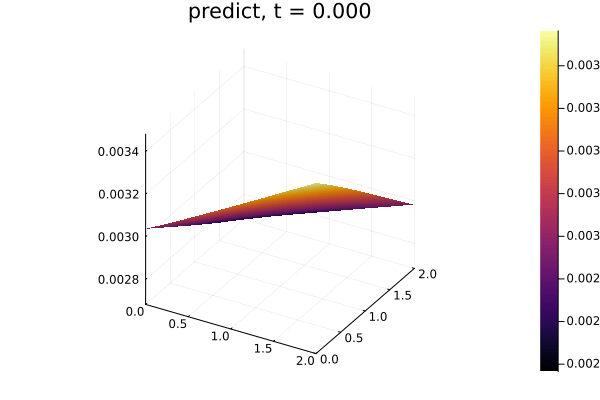Thanks in Advance! 1 Like It seems strange that you use Dx(ccDx(…)) and not ccDxx(…) Thank you, @Knud_Sorensen , for the question. It’s a precursor to the fact that C will be varying with X and Y in the future. And if it will be - that’s how it’s going to look. Do you think that this might be a problem? I do want to hear that I setup the problem wrong - since that restores my trust in NeuralPDE.jl… For example, I’m not sure about this line: Dt(u(t_min,x,y)) ~ 0.0 - is it doing anything at all? Should I replace it with Dt(u(t,x,y))(t_min,x,y) ~ 0.0. My physics understanding suggest that I should solve this: \begin{split}\begin{split} \frac{\partial^2 u}{\partial t^2} &= \frac{\partial}{\partial x}(c^2\frac{\partial u}{\partial x}) + \frac{\partial}{\partial y}(c^2\frac{\partial u}{\partial y}) \\ \frac{\partial u}{\partial t} - c\frac{\partial u}{\partial x} &= 0,\quad \mbox{ at } x = x_0 \\ \frac{\partial u}{\partial t} + c\frac{\partial u}{\partial x} &= 0,\quad \mbox{ at } x = x_e \\ \frac{\partial u}{\partial t} - c\frac{\partial u}{\partial y} &= 0,\quad \mbox{ at } y = y_0 \\ \frac{\partial u}{\partial t} + c\frac{\partial u}{\partial y} &= 0,\quad \mbox{ at } y = y_e \\ u(t_0,x,y) &= (x-x_{center}+0.2) \cdot e^{-12 \cdot ((x-x_{center})^2+(y-y_{center})^2)} \\ \frac{\partial u}{\partial t} &= 0,\quad \mbox{ at } t = t_0 \\ \end{split}\end{split} I don’t know if I formulated it right, though. Try to change it and see what happens. I also a newbie to working with differential equations in julia. then you put in the wrong boundary conditions. \begin{split}\begin{split}u - c\frac{\partial u}{\partial x} &= 0,\quad \mbox{ at } x = x_0 \ u + c\frac{\partial u}{\partial x} &= 0,\quad \mbox{ at } x = x_e \ u - c\frac{\partial u}{\partial y} &= 0,\quad \mbox{ at } y = y_0 \ u + c\frac{\partial u}{\partial y} &= 0,\quad \mbox{ at } y = y_e \ \end{split}\end{split} Sorry, don’t know how to get it rendered. Just put two dollar signs around the equations: \begin{split}\begin{split} u - c\frac{\partial u}{\partial x} &= 0,\quad \mbox{ at } x = x_0 \\ u + c\frac{\partial u}{\partial x} &= 0,\quad \mbox{ at } x = x_e \\ u - c\frac{\partial u}{\partial y} &= 0,\quad \mbox{ at } y = y_0 \\ u + c\frac{\partial u}{\partial y} &= 0,\quad \mbox{ at } y = y_e \\ \end{split}\end{split} Yes - my bad - was during a meeting when I typed it… Thank you for the correction. 1 Like Whom should I ask for help here - I’m clearly missing something and it feels that if an experienced person would look at it - he/she would immediately be able to tell what is going on. At this point - I’m really lost… Did you fix the boundary conditions in your code ?? What result do you get then ? I can see you went back and corrected your latex, but I think your latex was correct, and your code was wrong. 1 Like Yes! Looking into it… Well, I feel likenow. Just rerun it - still wrong. Here is the new code. using NeuralPDE, Lux, LuxCUDA, Random, ComponentArrays, CUDA using Optimization using OptimizationOptimisers import ModelingToolkit: Interval device = gpu_device() println("Setting Parameters") @parameters t x y @variables u(..) Dx = Differential(x) Dy = Differential(y) Dxx = Differential(x)^2 Dyy = Differential(y)^2 Dt = Differential(t) Dtt = Differential(t)^2 t_min = 0.0 t_max = 2.0 x_min = 0.0 x_max = 2.0 x_center = (x_min + x_max)/2 y_min = 0.0 y_max = 2.0 y_center = (y_min + y_max)/2 c = 1.0 first_maxiter = 250 retrain_maxiter = first_maxiter eq_scale=1000 bc_scale=1000 ic_scale=1000 println("Setting PDE") # 2D PDE eq = eq_scale*Dtt(u(t, x, y)) ~ eq_scale*(Dx(c*c*Dx(u(t, x, y))) + Dy(c*c*Dy(u(t, x, y)))) println("Setting IC and BC") open_bc = [ bc_scale*Dt(u(t,x_min,y)) ~ bc_scale*c*Dx(u(t,x_min,y)), bc_scale*Dt(u(t,x_max,y)) ~ -bc_scale*c*Dx(u(t,x_max,y)), bc_scale*Dt(u(t,x,y_min)) ~ bc_scale*c*Dx(u(t,x,y_min)), bc_scale*Dt(u(t,x,y_max)) ~ -bc_scale*c*Dx(u(t,x,y_max)) ] # impulse source IC ic_func(x,y) = (x-x_center+0.2)*exp(-12*((x-x_center)^2+(y-y_center)^2)) ic = [ ic_scale*u(t_min,x,y) ~ ic_scale*ic_func(x, y), ic_scale*Dt(u(t_min,x,y)) ~ 0.0 ] all_conditions = append!(open_bc, ic) println("Setting Space and Domain") # Space and time domains domains = [t ∈ Interval(t_min, t_max), x ∈ Interval(x_min, x_max), y ∈ Interval(y_min, y_max)] println("Setting NN") # Neural network inner = 25 chain = Chain(Dense(3, inner, Lux.σ), Dense(inner, inner, Lux.σ), Dense(inner, inner, Lux.σ), Dense(inner, inner, Lux.σ), Dense(inner, 1)) println("Prep PINN and cast to F64 GPU") strategy = GridTraining(0.05) ps, st = Lux.setup(Random.default_rng(), chain) ps = ps |> ComponentArray |> device .|> Float64 println("Setting discretization using PINN") discretization = PhysicsInformedNN(chain, strategy, init_params = ps) println("Putting it all together into PDESystem") @named pde_system = PDESystem(eq, all_conditions, domains, [t, x, y], [u(t, x, y)]) problem = discretize(pde_system, discretization) symprob = symbolic_discretize(pde_system, discretization) println("Start to solve using Optimization.Adam for 2500 max iterations") callback = function (p, l) println("Current loss is:$l")
return false
end

res = Optimization.solve(problem, Adam(0.1); callback = callback, maxiters = first_maxiter)

println("Remake a problem with warmed-up approximation from the above iterations")
prob = remake(problem, u0 = res.u)
res = Optimization.solve(problem, Adam(0.01); callback = callback, maxiters = retrain_maxiter)

println("Inspect the solution")

phi = discretization.phi
ts, xs, ys = [infimum(d.domain):0.1:supremum(d.domain) for d in domains]
#u_real = [analytic_sol_func(t, x, y) for t in ts for x in xs for y in ys]
u_predict = [first(Array(phi(device([t, x, y]), res.u))) for t in ts for x in xs for y in ys]

using Plots
using Printf

function plot_(res)
# Animate
anim = @animate for (i, t) in enumerate(t_min:0.05:t_max)
@info "Animating frame $i..." #u_real = reshape([analytic_sol_func(t, x, y) for x in xs for y in ys], # (length(xs), length(ys))) u_predict = reshape([first(Array(phi(device([t, x, y]), res.u))) for x in xs for y in ys], length(xs), length(ys)) #u_error = abs.(u_predict .- u_real) title = @sprintf("predict, t = %.3f", t) p1 = plot(xs, ys, u_predict, st = :surface, label = "", title = title) #title = @sprintf("real") #p2 = plot(xs, ys, u_real, st = :surface, label = "", title = title) #title = @sprintf("error") #p3 = plot(xs, ys, u_error, st = :contourf, label = "", title = title) plot(p1, #p2, #p3 ) end gif(anim, "3pde.gif", fps = 10) end println("First plot") plot_(res) println("Look at '3pde.gif'") function plot_wave(res) anim = @animate for (i,t) in enumerate(t_min:0.05:t_max) @info "Animating frame$i..."
u_predict = reshape([Array(phi(device([t, x, y]), res.u)) for x in xs for y in ys],
length(xs), length(ys))
#U,_ = unpack(sol(t))
surface(xs,ys,u_predict,layout=(1,2),size=(640,320),
xlabel="x",ylabel="y",
#zaxis=((-0.1,0.1),"u(x,y)"),
zlabel="u(x,y)",
color=:redsblues,alpha=0.66,
#clims=(-0.1,0.1),
colorbar=:none,
title="Wave equation",dpi=100 )
contour!(xs,ys,u_predict,levels=24,aspect_ratio=1,
subplot=2,
xlabel="x",ylabel="y",
color=:redsblues,
#clims=(-0.1,0.1),
colorbar=:none,
title=@sprintf("t = %.2f",t) )

end
closeall()
gif(anim, "wave_pde.gif", fps = 10)
end

println("Second plot")
plot_wave(res)

println("Look at 'wave_pde.gif'")


Any errors ? What does the new plot look like ?

No errors, @Knud_Sorensen .and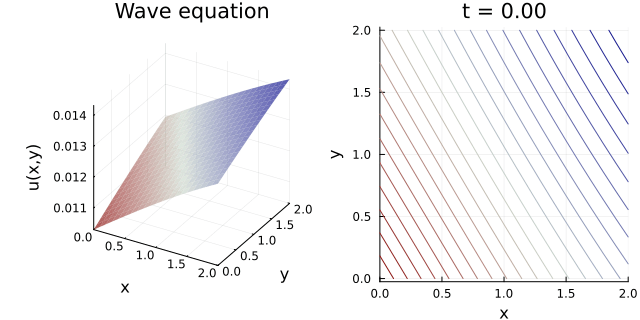How low does the loss go ?

The low goes as low as 1735 - please note that all constraints are scaled by 1000 - which is quite big.
If I set the scalers to 1.0 - then the error goes down to 0.001733 and this is how the gifs are looking.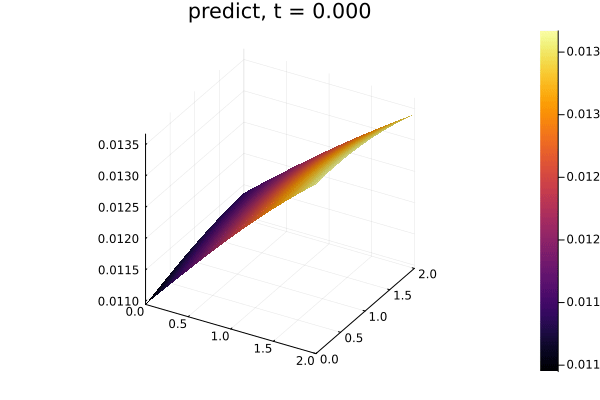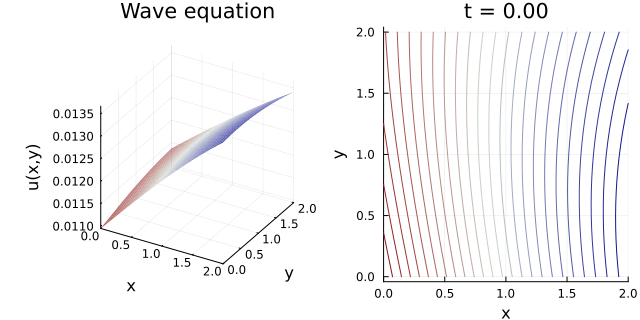So, it’s ether solves properly and I’m just not visualizing it well - or it’s just something else which I don’t understand.

Your program prints that it uses 2500 iterations
But in reality, it only uses 500.
Maybe try to run it for the full 2500 and see what the loss comes to.

I have played around with your code ! Is this more what you are looking for ?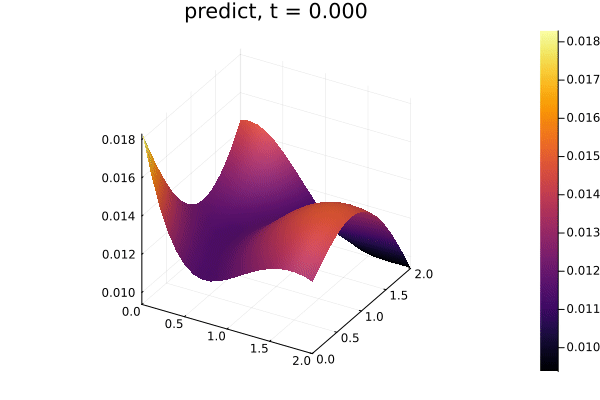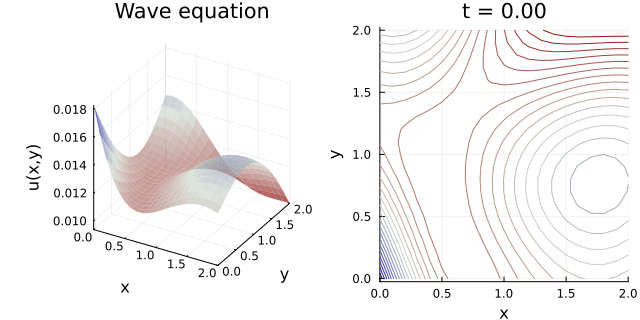1 Like